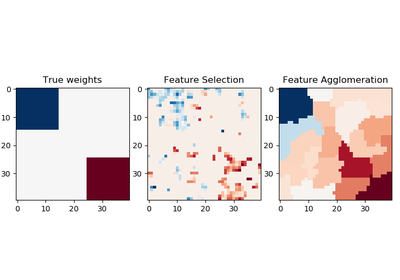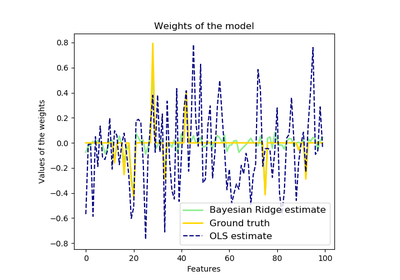# `sklearn.linear_model`.BayesianRidge¶

class `sklearn.linear_model.``BayesianRidge`(n_iter=300, tol=0.001, alpha_1=1e-06, alpha_2=1e-06, lambda_1=1e-06, lambda_2=1e-06, compute_score=False, fit_intercept=True, normalize=False, copy_X=True, verbose=False)[source]

Bayesian ridge regression

Fit a Bayesian ridge model and optimize the regularization parameters lambda (precision of the weights) and alpha (precision of the noise).

Read more in the User Guide.

Parameters: n_iter : int, optional Maximum number of iterations. Default is 300. tol : float, optional Stop the algorithm if w has converged. Default is 1.e-3. alpha_1 : float, optional Hyper-parameter : shape parameter for the Gamma distribution prior over the alpha parameter. Default is 1.e-6 alpha_2 : float, optional Hyper-parameter : inverse scale parameter (rate parameter) for the Gamma distribution prior over the alpha parameter. Default is 1.e-6. lambda_1 : float, optional Hyper-parameter : shape parameter for the Gamma distribution prior over the lambda parameter. Default is 1.e-6. lambda_2 : float, optional Hyper-parameter : inverse scale parameter (rate parameter) for the Gamma distribution prior over the lambda parameter. Default is 1.e-6 compute_score : boolean, optional If True, compute the objective function at each step of the model. Default is False fit_intercept : boolean, optional whether to calculate the intercept for this model. If set to false, no intercept will be used in calculations (e.g. data is expected to be already centered). Default is True. normalize : boolean, optional, default False This parameter is ignored when `fit_intercept` is set to False. If True, the regressors X will be normalized before regression by subtracting the mean and dividing by the l2-norm. If you wish to standardize, please use `sklearn.preprocessing.StandardScaler` before calling `fit` on an estimator with `normalize=False`. copy_X : boolean, optional, default True If True, X will be copied; else, it may be overwritten. verbose : boolean, optional, default False Verbose mode when fitting the model. coef_ : array, shape = (n_features) Coefficients of the regression model (mean of distribution) alpha_ : float estimated precision of the noise. lambda_ : float estimated precision of the weights. sigma_ : array, shape = (n_features, n_features) estimated variance-covariance matrix of the weights scores_ : float if computed, value of the objective function (to be maximized)

Notes

For an example, see examples/linear_model/plot_bayesian_ridge.py.

References

D. J. C. MacKay, Bayesian Interpolation, Computation and Neural Systems, Vol. 4, No. 3, 1992.

R. Salakhutdinov, Lecture notes on Statistical Machine Learning, http://www.utstat.toronto.edu/~rsalakhu/sta4273/notes/Lecture2.pdf#page=15 Their beta is our `self.alpha_` Their alpha is our `self.lambda_`

Examples

```>>> from sklearn import linear_model
>>> clf = linear_model.BayesianRidge()
>>> clf.fit([[0,0], [1, 1], [2, 2]], [0, 1, 2])
...
BayesianRidge(alpha_1=1e-06, alpha_2=1e-06, compute_score=False,
copy_X=True, fit_intercept=True, lambda_1=1e-06, lambda_2=1e-06,
n_iter=300, normalize=False, tol=0.001, verbose=False)
>>> clf.predict([[1, 1]])
array([ 1.])
```

Methods

 `fit`(X, y) Fit the model `get_params`([deep]) Get parameters for this estimator. `predict`(X[, return_std]) Predict using the linear model. `score`(X, y[, sample_weight]) Returns the coefficient of determination R^2 of the prediction. `set_params`(**params) Set the parameters of this estimator.
`__init__`(n_iter=300, tol=0.001, alpha_1=1e-06, alpha_2=1e-06, lambda_1=1e-06, lambda_2=1e-06, compute_score=False, fit_intercept=True, normalize=False, copy_X=True, verbose=False)[source]
`fit`(X, y)[source]

Fit the model

Parameters: X : numpy array of shape [n_samples,n_features] Training data y : numpy array of shape [n_samples] Target values. Will be cast to X’s dtype if necessary self : returns an instance of self.
`get_params`(deep=True)[source]

Get parameters for this estimator.

Parameters: deep : boolean, optional If True, will return the parameters for this estimator and contained subobjects that are estimators. params : mapping of string to any Parameter names mapped to their values.
`predict`(X, return_std=False)[source]

Predict using the linear model.

In addition to the mean of the predictive distribution, also its standard deviation can be returned.

Parameters: X : {array-like, sparse matrix}, shape = (n_samples, n_features) Samples. return_std : boolean, optional Whether to return the standard deviation of posterior prediction. y_mean : array, shape = (n_samples,) Mean of predictive distribution of query points. y_std : array, shape = (n_samples,) Standard deviation of predictive distribution of query points.
`score`(X, y, sample_weight=None)[source]

Returns the coefficient of determination R^2 of the prediction.

The coefficient R^2 is defined as (1 - u/v), where u is the residual sum of squares ((y_true - y_pred) ** 2).sum() and v is the total sum of squares ((y_true - y_true.mean()) ** 2).sum(). The best possible score is 1.0 and it can be negative (because the model can be arbitrarily worse). A constant model that always predicts the expected value of y, disregarding the input features, would get a R^2 score of 0.0.

Parameters: X : array-like, shape = (n_samples, n_features) Test samples. y : array-like, shape = (n_samples) or (n_samples, n_outputs) True values for X. sample_weight : array-like, shape = [n_samples], optional Sample weights. score : float R^2 of self.predict(X) wrt. y.
`set_params`(**params)[source]

Set the parameters of this estimator.

The method works on simple estimators as well as on nested objects (such as pipelines). The latter have parameters of the form `<component>__<parameter>` so that it’s possible to update each component of a nested object.

Returns: self :

## Examples using `sklearn.linear_model.BayesianRidge`¶Feature agglomeration vs. univariate selectionBayesian Ridge Regression